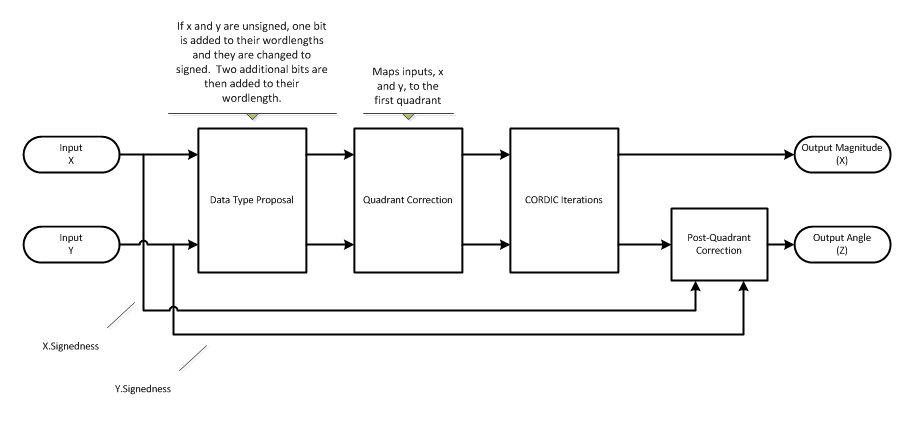# cordicatan2

## Syntax

``theta = cordicatan2(y,x)``
``theta = cordicatan2(y,x,niters)``

## Description

example

````theta = cordicatan2(y,x)` computes the four quadrant arctangent of `y` and `x` using a CORDIC algorithm approximation.```
````theta = cordicatan2(y,x,niters)` performs `niters` iterations of the algorithm.```

## Examples

collapse all

Define floating-point Cartesian coordinates.

```y = 0.5; x = -0.5;```

Use `cordicatan2` to compute floating-point CORDIC arctangent. Compare the result to the arctangent computed using `atan2`.

`theta_cdat2_float = cordicatan2(y,x)`
```theta_cdat2_float = 2.3562 ```
`theta_atan2_float = atan2(y,x)`
```theta_atan2_float = 2.3562 ```

Define fixed-point Cartesian coordinates.

```y = fi(0.5,1,16,15); x = fi(-0.5,1,16,15);```

Use `cordicatan2` to compute fixed-point CORDIC arctangent. Compare the result to the arctangent computed using `atan2`.

`theta_cdat2_fixpt = cordicatan2(y,x)`
```theta_cdat2_fixpt = 2.3562 DataTypeMode: Fixed-point: binary point scaling Signedness: Signed WordLength: 16 FractionLength: 13 ```
`theta_atan2_fixpt = atan2(y,x)`
```theta_atan2_fixpt = 2.3562 DataTypeMode: Fixed-point: binary point scaling Signedness: Signed WordLength: 16 FractionLength: 13 ```

## Input Arguments

collapse all

Cartesian y-coordinate, specified as a scalar, vector, matrix, or multidimensional array.

`y` and `x` must be the same size. If they are not the same size, at least one value must be a scalar value. `y` and `x` must have the same data type.

Data Types: `single` | `double` | `int8` | `int16` | `int32` | `int64` | `uint8` | `uint16` | `uint32` | `uint64` | `fi`
Complex Number Support: Yes

Cartesian x-coordinate, specified as a scalar, vector, matrix, or multidimensional array.

`y` and `x` must be the same size. If they are not the same size, at least one value must be a scalar value. `y` and `x` must have the same data type.

Data Types: `single` | `double` | `int8` | `int16` | `int32` | `int64` | `uint8` | `uint16` | `uint32` | `uint64` | `fi`
Complex Number Support: Yes

Number of iterations of CORDIC algorithm, specified as a positive, integer-valued scalar.

Increasing the number of iterations can produce more accurate results, but also increases the expense of the computation and adds latency.

If you do not specify `niters`, or if you specify a value that is too large, the algorithm uses a maximum value. For fixed-point operation, the maximum number of iterations is one less than the word length of `y` or `x`. For floating-point operation, the maximum value is 52 for double or 23 for single.

Data Types: `single` | `double` | `int8` | `int16` | `int32` | `int64` | `uint8` | `uint16` | `uint32` | `uint64` | `fi`

## Output Arguments

collapse all

Arctangent value in the range [-pi, pi] radians, returned as a scalar, vector, matrix, or multidimensional array.

If `y` and `x` are floating-point numbers, then `theta` has the same data type as `y` and `x`. Otherwise, `theta` is a fixed-point data type with the same word length as `y` and `x` and with a best-precision fraction length for the [-pi, pi] range.

## Algorithms

collapse all

### CORDIC

CORDIC is an acronym for COordinate Rotation DIgital Computer. The Givens rotation-based CORDIC algorithm is one of the most hardware-efficient algorithms available because it requires only iterative shift-add operations (see References). The CORDIC algorithm eliminates the need for explicit multipliers. Using CORDIC, you can calculate various functions such as sine, cosine, arc sine, arc cosine, arc tangent, and vector magnitude. You can also use this algorithm for divide, square root, hyperbolic, and logarithmic functions.

Increasing the number of CORDIC iterations can produce more accurate results, but doing so increases the expense of the computation and adds latency.

### Signal Flow Diagram### CORDIC Vectoring KernelThe accuracy of the CORDIC kernel depends on the choice of initial values for X, Y, and Z. This algorithm uses the following initial values:

• X0 is initialized to the X input value

• Y0 is initialized to the Y input value

• Z0 is initialized `0`

### `fimath` Propagation Rules

CORDIC functions discard any local `fimath` attached to the input.

The CORDIC functions use their own internal `fimath` when performing calculations:

• `OverflowAction``Wrap`

• `RoundingMethod``Floor`

The output has no attached `fimath`.

## Version History

Introduced in R2011b